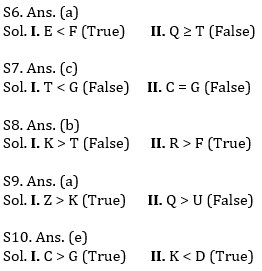Latest Banking jobs   »   Reasoning Ability Quiz For IBPS Clerk/NIACL...

# Reasoning Ability Quiz For IBPS Clerk/NIACL AO Prelims 2021- 3rd September

Directions (1-5): Study the information carefully and answer the questions given below.
Eight persons are sitting around a square table such that four of them sit at the corners and rest on the middle of the side. The persons sitting on the corners face opposite direction to that of the ones sitting on the middle of the side. The one sitting immediate left to D does not face outside. E and B are immediate left to each other. C faces A. F and D are immediate neighbors of each other. The one sitting 5th right to the one who is 3rd left to A does not sit at the corner. G sits 3rd right to A. H sits opposite to E.

Q1. What is the position of C with respect to D?
(a) 3rd to the left
(b) 3rd to the right
(c) 4th to the left
(d) 5th to the right
(e) none of these

Q2. How many persons are sitting between A and F, when counted form the right of F?
(a) Two
(b) One
(c) Three
(d) Four
(e) None of these

Q3. Who among the following sits opposite to B?
(a) E
(b) F
(c) C
(d) A
(e) none of these

Q4. What is the position of H with respect to E?
(a) 3rd to the left
(b) 3rd to the right
(c) 4th to the left
(d) 5th to the right
(e) none of these

Q5. Four of following form a group find the one that does not belong to that group?
(a) E
(b) F
(c) C
(d) B
(e) A

Directions (6-10): In these questions, relationship between different elements is show in the statements. The statements are followed by conclusions. Study the conclusions based on the given statements and select the appropriate answer:
(a) If only conclusion I follows.
(b) If only conclusion II follows.
(c) If either conclusion I or II follows.
(d) If neither conclusion I nor II follows.
(e) If both conclusions I and II follow.

Q6. Statements: F > R ≥ T = E > W ≤ Q
Conclusions: I. E < F II. Q ≥ T

Q7. Statements: W = G ≥ H ≥ T = C ≤ V ≤ B
Conclusions: I. T < G II. C = G

Q8. Statements: K > I ≥ G > F ≤ T < R
Conclusions: I. K > T II. R > F

Q9. Statements: Q > O ≥ P = K; O < U ≤ Z
Conclusions: I. Z > K II. Q > U

Q10. Statements: K < G ≤ D < C > R ≤ Y
Conclusions: I. C > G II. K < D

Directions (11-15): Answer these questions referring to the letter sequence given below:

O P H Z A R S N I J K U V G Q Y B F E L M T W X D C

Q11. If letters of the above given series are written in reverse order, then which letter will be third to the left of eighteenth letter from right?
(a) Z
(b) M
(c) I
(d) L
(e) None of these

Q12. Which of the following letter is 3rd to left of 6th from left?
(a) S
(b) A
(c) I
(d) F
(e) H

Q13. Which of the following is the fifth to the right of thirteenth letter from left?
(a) U
(b) J
(c) S
(d) Z
(e) None of these

Q14. Which of the following is 5th to right of 3rd from left?
(a) C
(b) Q
(c) K
(d) Y
(e) N

Q15. Which of the following letter is 4th to right of 8th from right?
(a) W
(b) T
(c) X
(d) E
(e) P

SolutionsS11. Ans.(b)

S12. Ans.(e)

S13. Ans.(e)

S14. Ans.(e)

S15. Ans.(a)

Click Here to Register for Bank Exams 2021 Preparation Material#### Congratulations!Download Hindu Review of October 2021: Free PDF